# RS Aggarwal Solutions for Class 8 Maths Chapter 10 - Profit and Loss Exercise 10A

Download RS Aggarwal Solutions for Class 8 Maths Chapter 10- Exercise 10A, Profit and Loss from the links provided below. BYJU’S expert team have solved the RS Aggarwal Solutions to ensure that the students are thorough with their fundamentals by practising the solutions. In Exercise 10A of RS Aggarwal Class 8 Maths, we shall see about the cost price, selling price, profit or gain and loss related questions.

## Download PDF of RS Aggarwal Solutions for Class 8 Maths Chapter 10- Profit and Loss Exercise 10A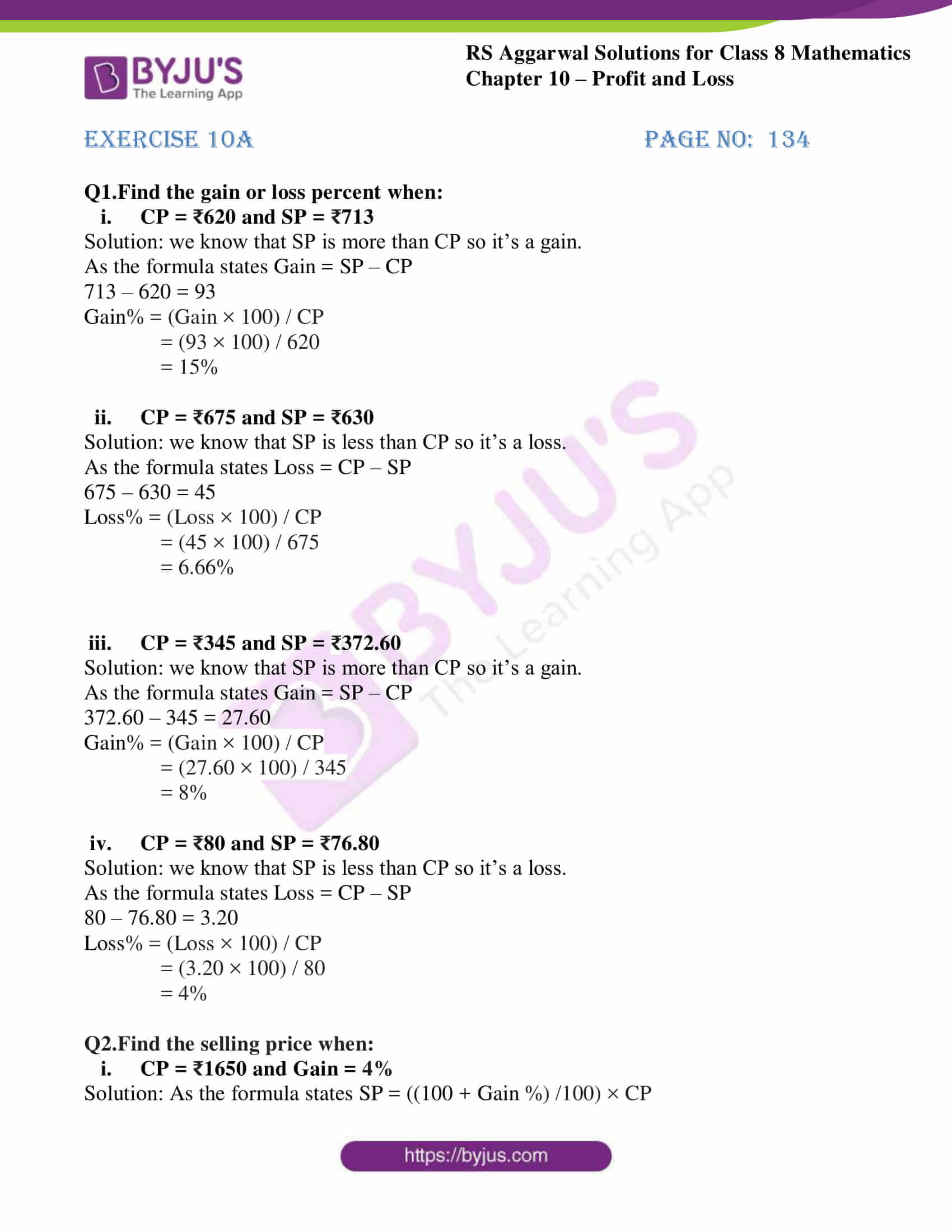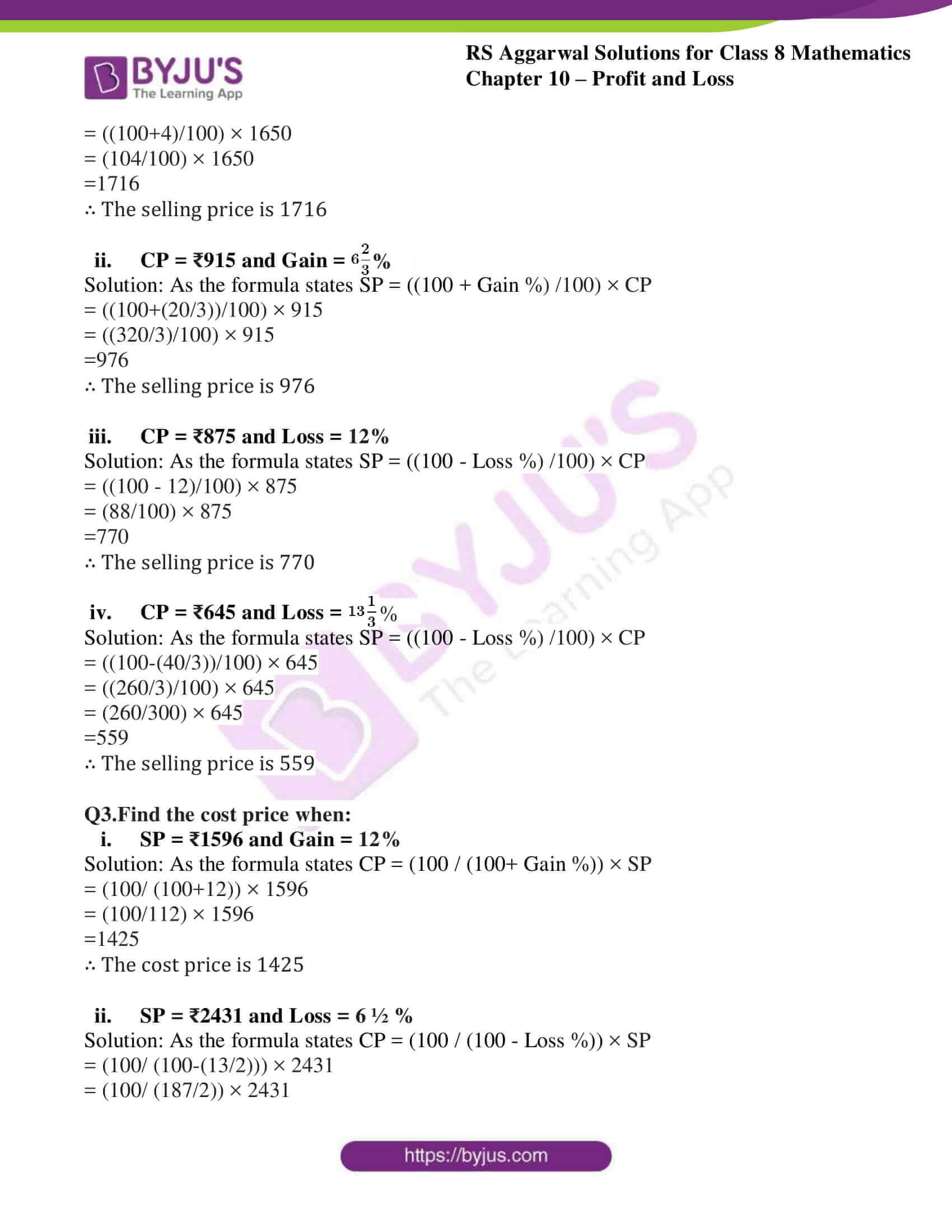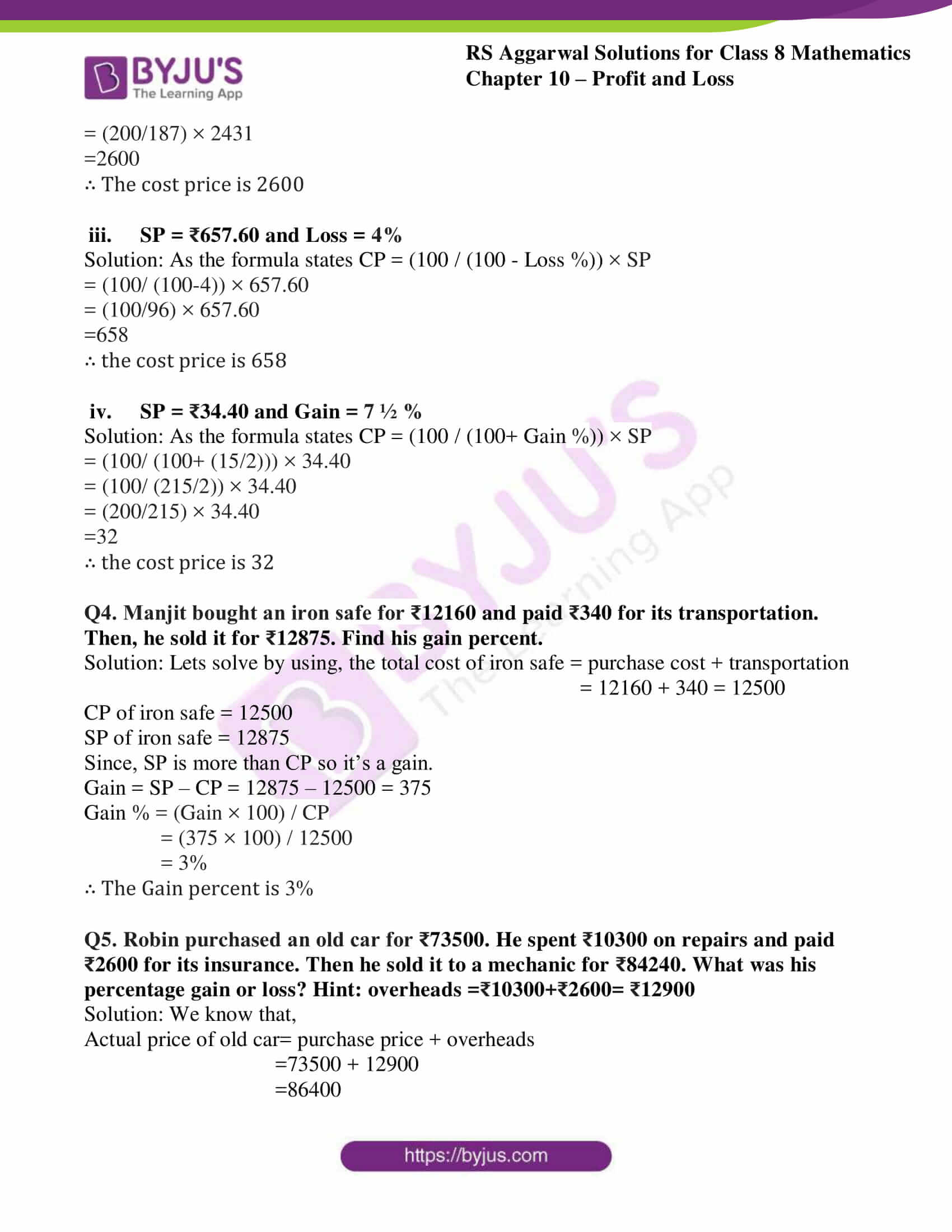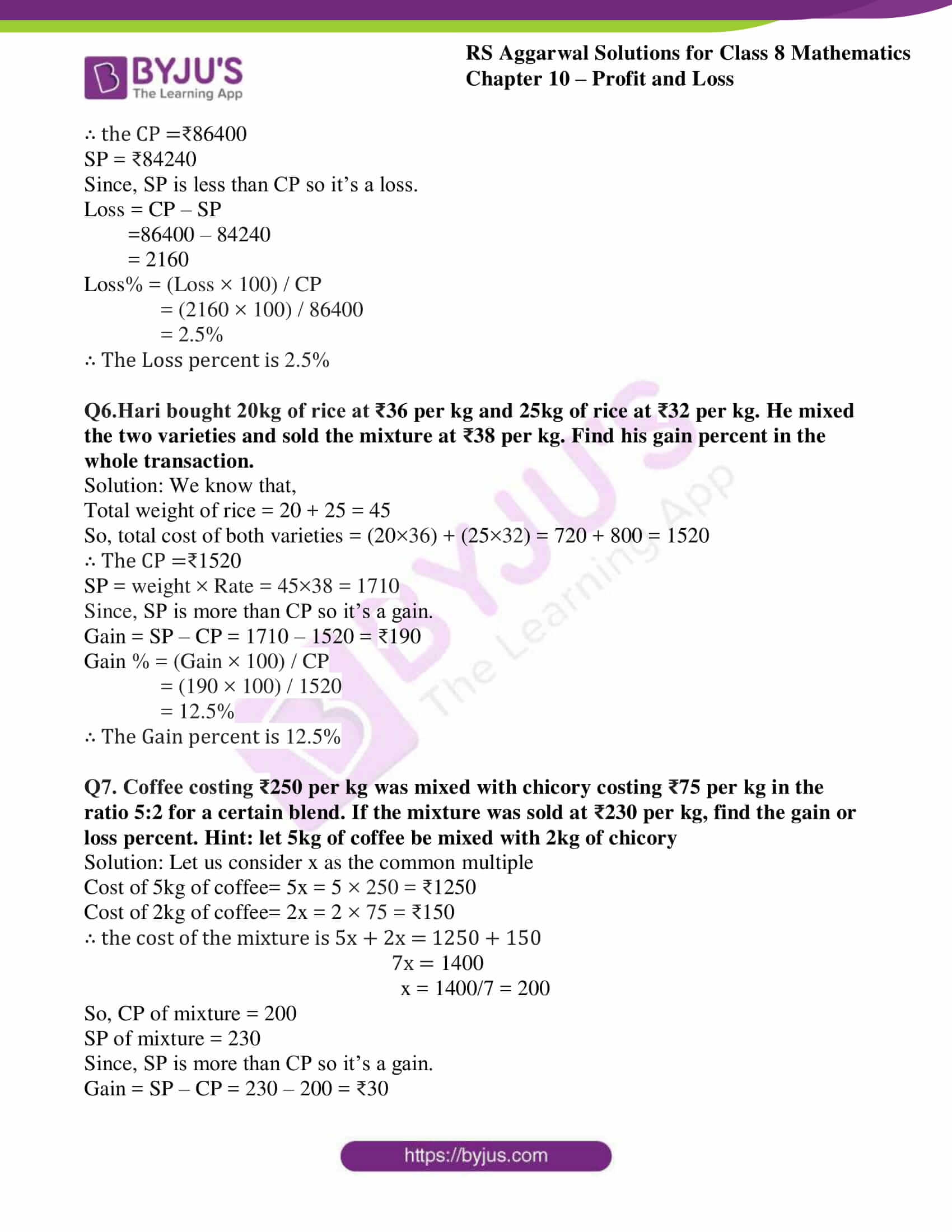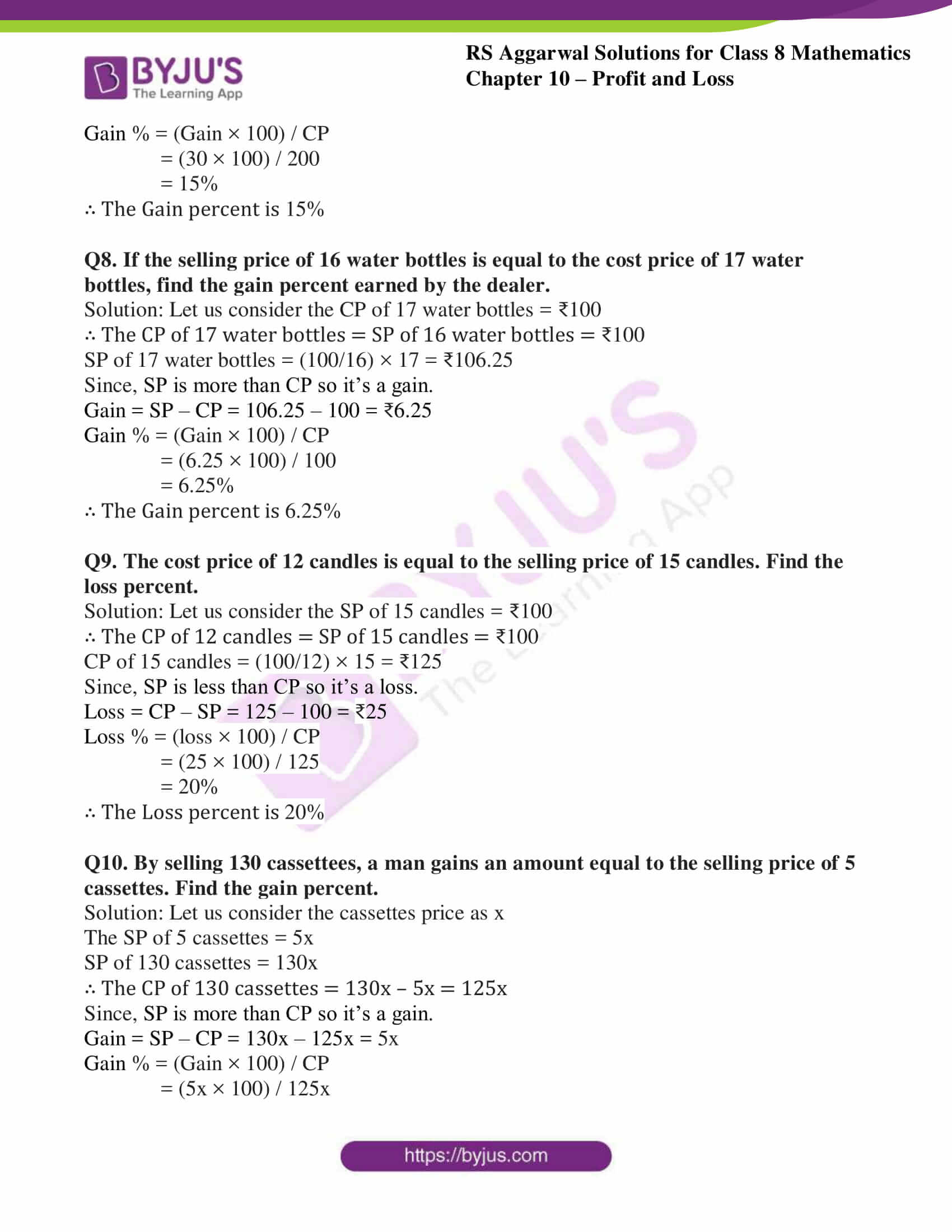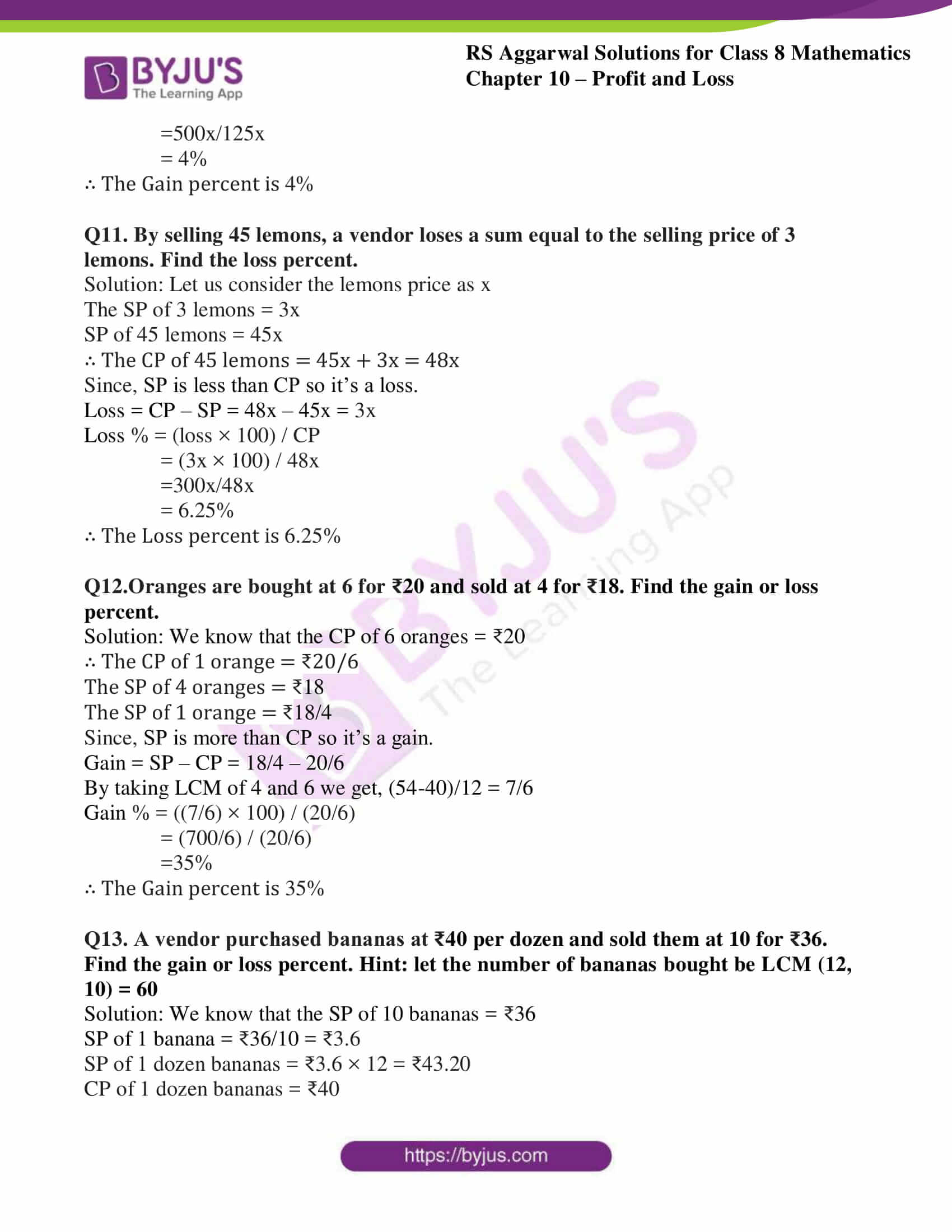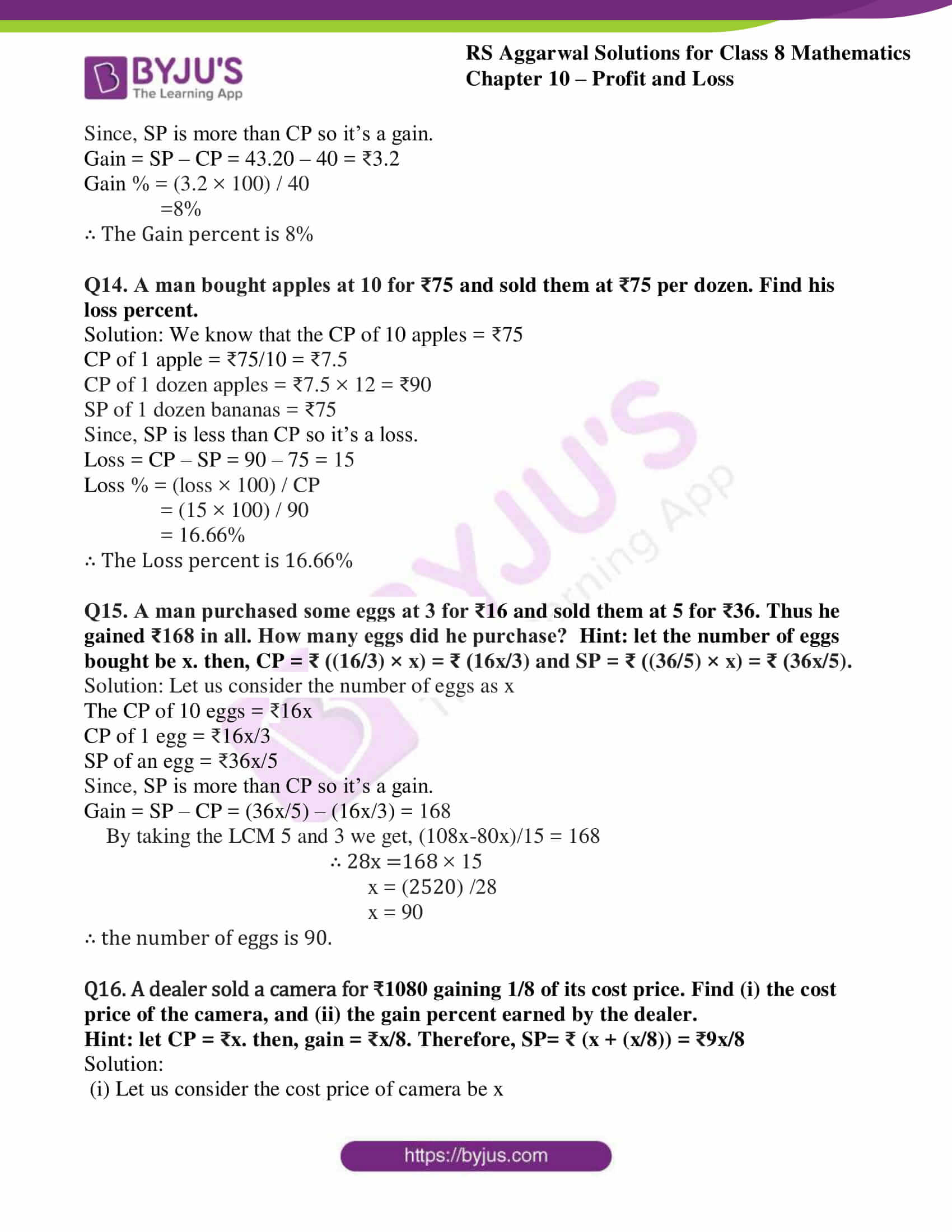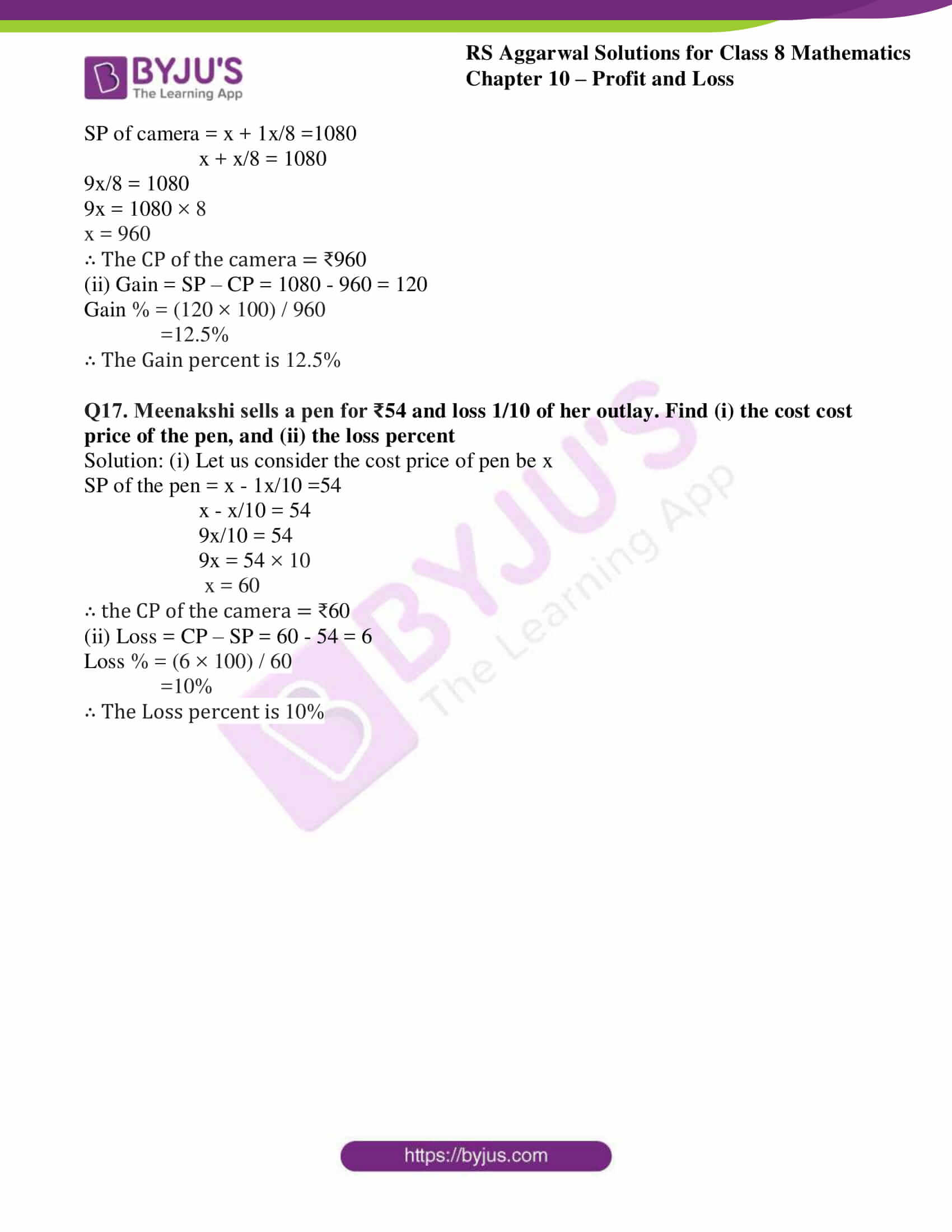### Access Answers to RS Aggarwal Solutions for Class 8 Maths Chapter 10- Profit and Loss Exercise 10A

Q1.Find the gain or loss percent when:

i CP = ₹620 and SP = ₹713

Solution: we know that SP is more than CP so it’s a gain.As the formula states Gain = SP – CP

713 – 620 = 93

Gain% = (Gain × 100) / CP

= (93 × 100) / 620

= 15%

ii CP = ₹675 and SP = ₹630

Solution: we know that SP is less than CP so it’s a loss.As the formula states Loss = CP – SP

675 – 630 = 45

Loss% = (Loss × 100) / CP

= (45 × 100) / 675

= 6.66%

iii CP = ₹345 and SP = ₹372.60

Solution: we know that SP is more than CP so it’s a gain.As the formula states Gain = SP – CP

372.60 – 345 = 27.60

Gain% = (Gain × 100) / CP

= (27.60 × 100) / 345

= 8%

iv CP = ₹80 and SP = ₹76.80

Solution: we know that SP is less than CP so it’s a loss.As the formula states Loss = CP – SP

80 – 76.80 = 3.20

Loss% = (Loss × 100) / CP

= (3.20 × 100) / 80

= 4%

Q2.Find the selling price when:

i CP = ₹1650 and Gain = 4%

Solution: As the formula states SP = ((100 + Gain %) /100) × CP= ((100+4)/100) × 1650

= (104/100) × 1650

=1716

∴ The selling price is 1716

ii CP = ₹915 and Gain = $6\frac{2}{3}$%

Solution: As the formula states SP = ((100 + Gain %) /100) × CP= ((100+(20/3))/100) × 915

= ((320/3)/100) × 915

=976

∴ The selling price is 976

iii CP = ₹875 and Loss = 12%

Solution: As the formula states SP = ((100 – Loss %) /100) × CP= ((100 – 12)/100) × 875

= (88/100) × 875

=770

∴ The selling price is 770

iv CP = ₹645 and Loss = $13\frac{1}{3}$%

Solution: As the formula states SP = ((100 – Loss %) /100) × CP= ((100-(40/3))/100) × 645

= ((260/3)/100) × 645

= (260/300) × 645

=559

∴ The selling price is 559

Q3.Find the cost price when:

i SP = ₹1596 and Gain = 12%

Solution: As the formula states CP = (100 / (100+ Gain %)) × SP= (100/ (100+12)) × 1596

= (100/112) × 1596

=1425

∴ The cost price is 1425

ii SP = ₹2431 and Loss = 6 ½ %

Solution: As the formula states CP = (100 / (100 – Loss %)) × SP= (100/ (100-(13/2))) × 2431

= (100/ (187/2)) × 2431

= (200/187) × 2431

=2600

∴ The cost price is 2600

iii SP = ₹657.60 and Loss = 4%

Solution: As the formula states CP = (100 / (100 – Loss %)) × SP= (100/ (100-4)) × 657.60

= (100/96) × 657.60

=658

∴ the cost price is 658

iv SP = ₹34.40 and Gain = 7 ½ %

Solution: As the formula states CP = (100 / (100+ Gain %)) × SP= (100/ (100+ (15/2))) × 34.40

= (100/ (215/2)) × 34.40

= (200/215) × 34.40

=32

∴ the cost price is 32

Q4. Manjit bought an iron safe for ₹12160 and paid ₹340 for its transportation. Then, he sold it for ₹12875. Find his gain percent.

Solution: Lets solve by using, the total cost of iron safe = purchase cost + transportation

= 12160 + 340 = 12500

CP of iron safe = 12500

SP of iron safe = 12875

Since, SP is more than CP so it’s a gain.

Gain = SP – CP = 12875 – 12500 = 375

Gain % = (Gain × 100) / CP

= (375 × 100) / 12500

= 3%

∴ The Gain percent is 3%

Q5. Robin purchased an old car for ₹73500. He spent ₹10300 on repairs and paid ₹2600 for its insurance. Then he sold it to a mechanic for ₹84240. What was his percentage gain or loss? Hint: overheads =₹10300+₹2600= ₹12900

Solution: We know that,

Actual price of old car= purchase price + overheads

=73500 + 12900

=86400

∴ the CP =₹86400

SP = ₹84240

Since, SP is less than CP so it’s a loss.

Loss = CP – SP

=86400 – 84240

= 2160

Loss% = (Loss × 100) / CP

= (2160 × 100) / 86400

= 2.5%

∴ The Loss percent is 2.5%

Q6.Hari bought 20kg of rice at ₹36 per kg and 25kg of rice at ₹32 per kg. He mixed the two varieties and sold the mixture at ₹38 per kg. Find his gain percent in the whole transaction.

Solution: We know that,

Total weight of rice = 20 + 25 = 45

So, total cost of both varieties = (20×36) + (25×32) = 720 + 800 = 1520

∴ The CP =₹1520

SP = weight × Rate = 45×38 = 1710

Since, SP is more than CP so it’s a gain.

Gain = SP – CP = 1710 – 1520 = ₹190

Gain % = (Gain × 100) / CP

= (190 × 100) / 1520

= 12.5%

∴ The Gain percent is 12.5%

Q7. Coffee costing ₹250 per kg was mixed with chicory costing ₹75 per kg in the ratio 5:2 for a certain blend. If the mixture was sold at ₹230 per kg, find the gain or loss percent. Hint: let 5kg of coffee be mixed with 2kg of chicory

Solution: Let us consider x as the common multiple

Cost of 5kg of coffee= 5x = 5 × 250 = ₹1250

Cost of 2kg of coffee= 2x = 2 × 75 = ₹150

∴ the cost of the mixture is 5x + 2x = 1250 + 150

7x = 1400

x = 1400/7 = 200

So, CP of mixture = 200

SP of mixture = 230

Since, SP is more than CP so it’s a gain.

Gain = SP – CP = 230 – 200 = ₹30

Gain % = (Gain × 100) / CP

= (30 × 100) / 200

= 15%

∴ The Gain percent is 15%

Q8. If the selling price of 16 water bottles is equal to the cost price of 17 water bottles, find the gain percent earned by the dealer.

Solution: Let us consider the CP of 17 water bottles = ₹100

∴ The CP of 17 water bottles = SP of 16 water bottles = ₹100

SP of 17 water bottles = (100/16) × 17 = ₹106.25

Since, SP is more than CP so it’s a gain.

Gain = SP – CP = 106.25 – 100 = ₹6.25

Gain % = (Gain × 100) / CP

= (6.25 × 100) / 100

= 6.25%

∴ The Gain percent is 6.25%

Q9. The cost price of 12 candles is equal to the selling price of 15 candles. Find the loss percent.

Solution: Let us consider the SP of 15 candles = ₹100

∴ The CP of 12 candles = SP of 15 candles = ₹100

CP of 15 candles = (100/12) × 15 = ₹125

Since, SP is less than CP so it’s a loss.

Loss = CP – SP = 125 – 100 = ₹25

Loss % = (loss × 100) / CP

= (25 × 100) / 125

= 20%

∴ The Loss percent is 20%

Q10. By selling 130 cassettees, a man gains an amount equal to the selling price of 5 cassettes. Find the gain percent.

Solution: Let us consider the cassettes price as x

The SP of 5 cassettes = 5x

SP of 130 cassettes = 130x

∴ The CP of 130 cassettes = 130x – 5x = 125x

Since, SP is more than CP so it’s a gain.

Gain = SP – CP = 130x – 125x = 5x

Gain % = (Gain × 100) / CP

= (5x × 100) / 125x

=500x/125x

= 4%

∴ The Gain percent is 4%

Q11. By selling 45 lemons, a vendor loses a sum equal to the selling price of 3 lemons. Find the loss percent.

Solution: Let us consider the lemons price as x

The SP of 3 lemons = 3x

SP of 45 lemons = 45x

∴ The CP of 45 lemons = 45x + 3x = 48x

Since, SP is less than CP so it’s a loss.

Loss = CP – SP = 48x – 45x = 3x

Loss % = (loss × 100) / CP

= (3x × 100) / 48x

=300x/48x

= 6.25%

∴ The Loss percent is 6.25%

Q12.Oranges are bought at 6 for ₹20 and sold at 4 for ₹18. Find the gain or loss percent.

Solution: We know that the CP of 6 oranges = ₹20

∴ The CP of 1 orange = ₹20/6

The SP of 4 oranges = ₹18

The SP of 1 orange = ₹18/4

Since, SP is more than CP so it’s a gain.

Gain = SP – CP = 18/4 – 20/6

By taking LCM of 4 and 6 we get, (54-40)/12 = 7/6

Gain % = ((7/6) × 100) / (20/6)

= (700/6) / (20/6)

=35%

∴ The Gain percent is 35%

Q13. A vendor purchased bananas at ₹40 per dozen and sold them at 10 for ₹36. Find the gain or loss percent. Hint: let the number of bananas bought be LCM (12, 10) = 60

Solution: We know that the SP of 10 bananas = ₹36

SP of 1 banana = ₹36/10 = ₹3.6

SP of 1 dozen bananas = ₹3.6 × 12 = ₹43.20

CP of 1 dozen bananas = ₹40

Since, SP is more than CP so it’s a gain.

Gain = SP – CP = 43.20 – 40 = ₹3.2

Gain % = (3.2 × 100) / 40

=8%

∴ The Gain percent is 8%

Q14. A man bought apples at 10 for ₹75 and sold them at ₹75 per dozen. Find his loss percent.

Solution: We know that the CP of 10 apples = ₹75

CP of 1 apple = ₹75/10 = ₹7.5

CP of 1 dozen apples = ₹7.5 × 12 = ₹90

SP of 1 dozen bananas = ₹75

Since, SP is less than CP so it’s a loss.

Loss = CP – SP = 90 – 75 = 15

Loss % = (loss × 100) / CP

= (15 × 100) / 90

= 16.66%

∴ The Loss percent is 16.66%

Q15. A man purchased some eggs at 3 for ₹16 and sold them at 5 for ₹36. Thus he gained ₹168 in all. How many eggs did he purchase? Hint: let the number of eggs bought be x. then, CP = ₹ ((16/3) × x) = ₹ (16x/3) and SP = ₹ ((36/5) × x) = ₹ (36x/5).

Solution: Let us consider the number of eggs as x

The CP of 10 eggs = ₹16x

CP of 1 egg = ₹16x/3

SP of an egg = ₹36x/5

Since, SP is more than CP so it’s a gain.

Gain = SP – CP = (36x/5) – (16x/3) = 168

By taking the LCM 5 and 3 we get, (108x-80x)/15 = 168

∴ 28x =168 × 15

x = (2520) /28

x = 90

∴ the number of eggs is 90.

Q16. A dealer sold a camera for ₹1080 gaining 1/8 of its cost price. Find (i) the cost price of the camera, and (ii) the gain percent earned by the dealer.

Hint: let CP = ₹x. then, gain = ₹x/8. Therefore, SP= ₹ (x + (x/8)) = ₹9x/8

Solution:

(i) Let us consider the cost price of camera be x

SP of camera = x + 1x/8 =1080

x + x/8 = 1080

9x/8 = 1080

9x = 1080 × 8

x = 960

∴ The CP of the camera = ₹960

(ii) Gain = SP – CP = 1080 – 960 = 120

Gain % = (120 × 100) / 960

=12.5%

∴ The Gain percent is 12.5%

Q17. Meenakshi sells a pen for ₹54 and loss 1/10 of her outlay. Find (i) the cost cost price of the pen, and (ii) the loss percent

Solution: (i) Let us consider the cost price of pen be x

SP of the pen = x – 1x/10 =54

x – x/10 = 54

9x/10 = 54

9x = 54 × 10

x = 60

∴ the CP of the camera = ₹60

(ii) Loss = CP – SP = 60 – 54 = 6

Loss % = (6 × 100) / 60

=10%

∴ The Loss percent is 10%

### Access other Exercises of RS Aggarwal Solutions for Class 8 Maths Chapter 10- Profit and Loss

Exercise 10B Solutions 14 Questions

Exercise 10C Solutions 11 Questions

Exercise 10D Solutions 21 Questions

## RS Aggarwal Solutions for Class 8 Maths Chapter 10- Profit and Loss Exercise 10A

Exercise 10A of RS Aggarwal Class 8, Profit and Loss. This exercise mainly deals with the cost price- The amount for which an article is bought; selling price- The amount for which an article is sold; profit- when selling price is more than the cost price then its a gain; loss- when selling price is less than the cost price then its a loss.

Students are suggested to practice the problems on a regular basis which will help them excel in their exams and increase their overall percentage. Practising as many times as possible helps in building time management skills and also boosts the confidence level to achieve high marks.